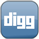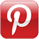### Drawing a CurvePreviously, we had looked at the basics of drawing on the canvas- setting line styles, gradients, and stroking lines. But drawing straight lines doesn't help us if we're looking to draw a curve. We'll look at the bezierCurveTo() and quadraticCurveTo() of the context object.

Bezier curves are determined by specifying one or two control points and a destination point. The call to quadraticCurveTo() takes a single control point, while bezierCurveTo() takes two.

context.bezierCurveTo(cp1x, cp1y, cp2x, cp2y, x, y);

The starting point for the curve is always determined by the current pen position, similar to how lineTo() works. The destination point is simply the ending point of where you are drawing the curve to. The control points determine the shape of the curve; they do so by determining where tangents to the curve at the starting point and destination points intersect. See an example below:

``` context.quadraticCurveTo(0, 200, 200, 200); ```